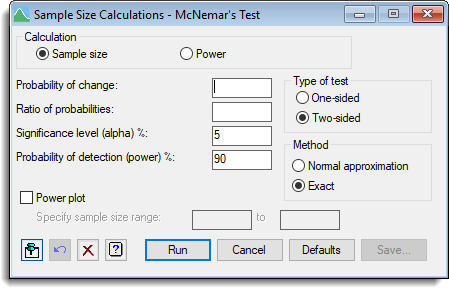1. Home
2. Sample Size Calculations – McNemar’s test

# Sample Size Calculations – McNemar’s test

Select menu: Stats | Sample Size | McNemar’s test

Use this to calculate the number of replicates (sample size) or the power required for a McNemar test.

Stats | Sample Size | McNemar’s test.
2. Fill in the fields as required then click Run.

After running the analysis you can save the results by clicking Save.The McNemar test is useful for analyzing studies where subjects are assessed before and after a treatment. The response on each occasion is assumed to be categorized by a factor with two levels, with level 1 usually representing a negative response, and level 2 a positive response. The test is based on a table giving the numbers of subjects giving each combination of responses over the two occasions.

## Calculation

Specifies the calculation to be carried out: Sample size, or Power.

## Probability of change

Specifies the overall probability of change, e.g. changes from positive to negative, or negative to positive.

## Ratio of probabilities

Specifies the ratio of the probabilities of the two types of change, e.g. ratio between changes from positive to negative versus negative to positive.

## Significance level (alpha)%

Specifies the significance level at which the response is to be tested.

## Probability of detection (power)%

For a sample size calculation this specifies the required probability for detection of the response (that is, the power of the test).

## Sample size

For a power calculation this specifies the sample size to be used to calculate the probability of detection (power).

## Power plot

When selected, produces a plot of power versus sample size. When Sample size is selected the plot is generated using 11 replication values centred around the required number of replicates for the requested power. When Power is selected you can specify a range of sample size values for the plot in the spaces provided.

## Type of test

Lets you choose either a one-sided or two-sided test.

## Method

Specifies the method for the test that is to be done. The Normal approximation setting relates to a test based on the Normal approximation, while the Exact assumes an exact test using the binomial distribution.

## Action IconsPin Controls whether to keep the dialog open when you click Run. When the pin is downthe dialog will remain open, otherwise when the pin is upthe dialog will close.Restore Restore names into edit fields and default settings.Clear Clear all fields and list boxes.Help Open the Help topic for this dialog.